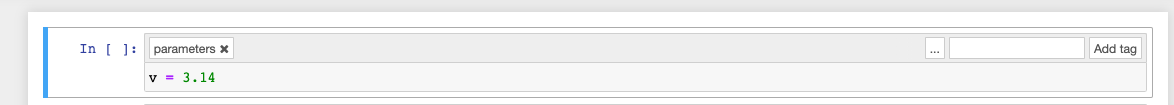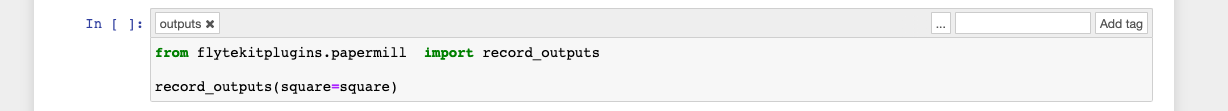In this example, we will show how to create a flyte task that runs a simple notebook, accepts one input variable, transforms it, and produces one output. This can be generalized to multiple inputs and outputs.

```import math
import os
import pathlib

from flytekit import kwtypes, task, workflow
```

## How to specify inputs and outputs#

1. After you are satisfied with the notebook, ensure that the first cell only has the input variables for the notebook. Now add the tag `parameters` for the first cell.1. Typically at the last cell of the notebook (which does not need to be the last cell), add a tag `outputs` for the intended cell.1. In a python file, create a new task at the `module` level. An example task is shown below:

```nb = NotebookTask(
name="simple-nb",
notebook_path=os.path.join(pathlib.Path(__file__).parent.absolute(), "nb_simple.ipynb"),
render_deck=True,
inputs=kwtypes(v=float),
outputs=kwtypes(square=float),
)
```

Note

• Note the notebook_path. This is the absolute path to the actual notebook.

• Note the inputs and outputs. The variable names match the variable names in the jupyter notebook.

• You can see the notebook on Flyte deck if `render_deck` is set to true.

You can definitely declare other tasks and seamlessly work with notebook tasks. The example below shows how to declare a task that accepts the squared value from the notebook and provides a sqrt:

```@task
return math.sqrt(f)
```

```@workflow
def nb_to_python_wf(f: float) -> float:
out = nb(v=f)
```if __name__ == "__main__":
One of them is the executed notebook (captured) and a rendered (HTML) of the executed notebook. In this case they are called `nb-simple-out.ipynb` and `nb-simple-out.html`, respectively.